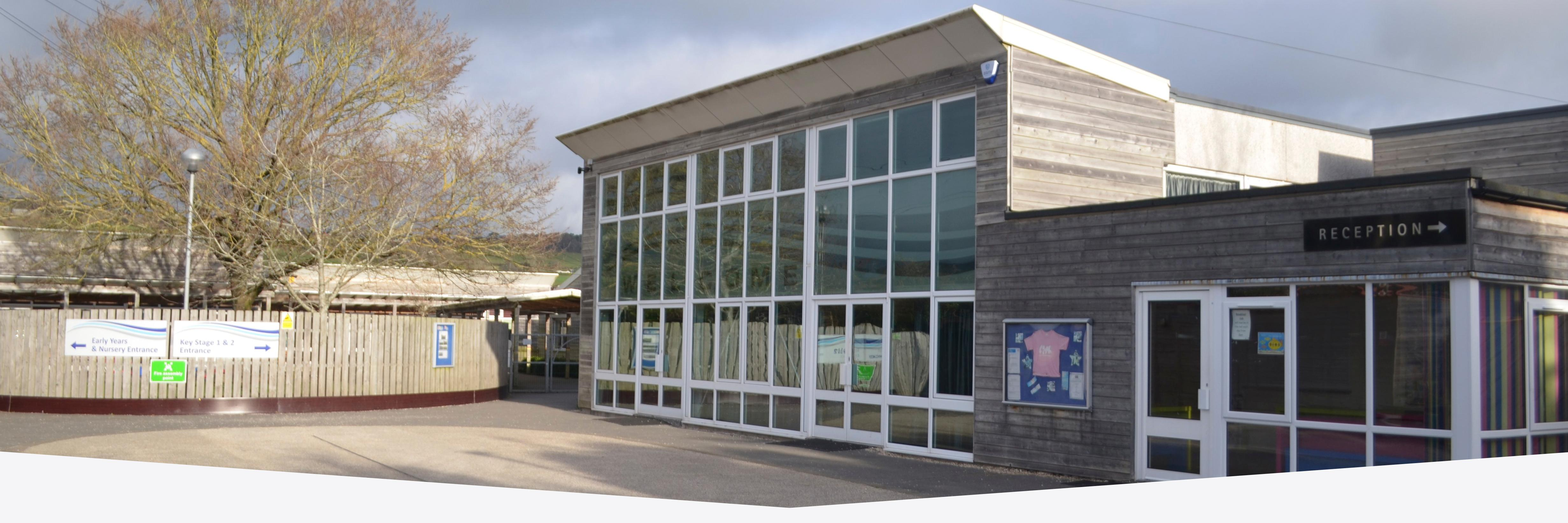# Solving one step multiplication

We are learning to (WALT) solve one step multiplication using concrete objects and an array

WB: 29/4/2019This week in Year 1, we have been learning how to multiply.

We began by looking at repeated addition and making equal groups of objects.

We then introduced the division symbol and used concrete objects and pictorial representations to solve simple calculations.

For week 2, we began using arrays to solve these.

We worked on the carpet as a class and discussed the language used when multiplying. Groups of/lots of/times/multiply. We used paint and cotton buds to make our arrays into our maths books.